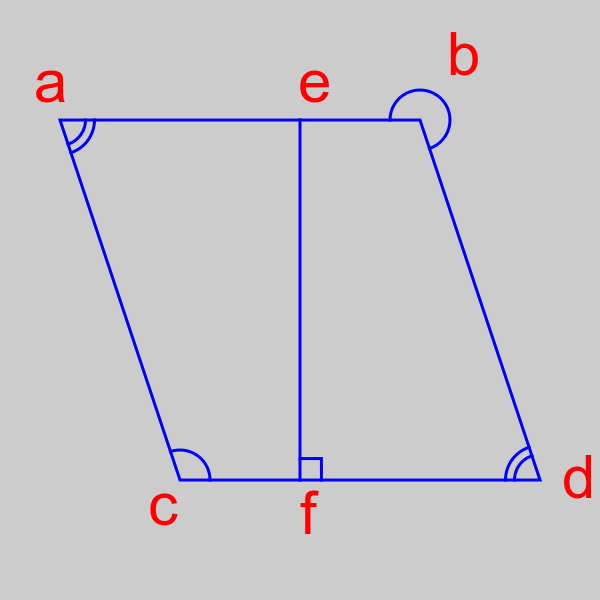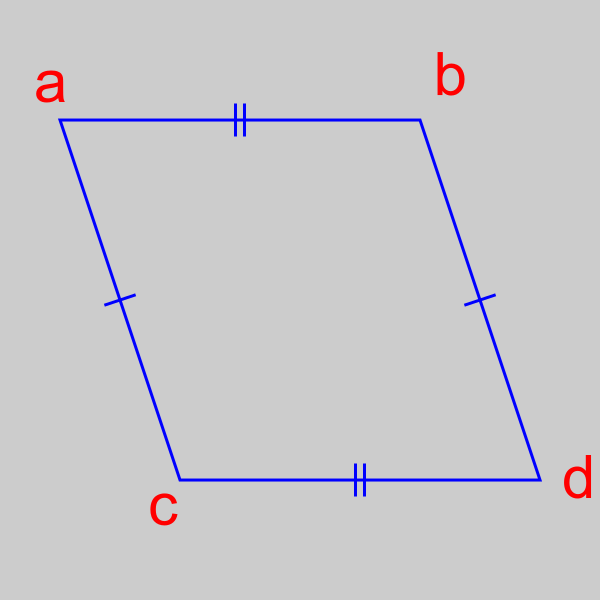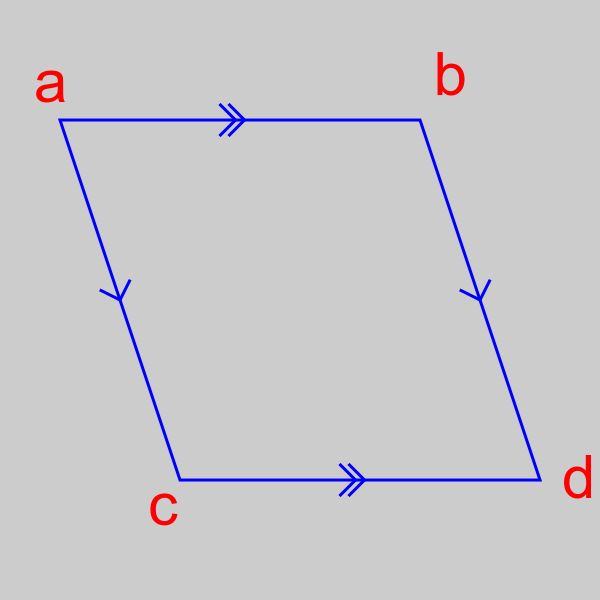# Geometric markers in generativepy

Martin McBride, 2022-02-01
Tags marker angle line length parallel
Categories generativepy generativepy tutorialThis tutorial shows how to add geometric makers in generativepy.

There are several different markers:

• AngleMarker marks an angle. Double and triple markers can be used to indicate that two angles are identical. The marker also provides a right angle style.
• Tickmarker marks a line with a single, double, or triple tick. This is used to indicate that two-line lengths are identical.
• Paramarker marks a line with single, double, or triple arrows. This is used to indicate that two-line lengths are parallel.

Each marker is a shape object that is drawn separately from the lines themselves, although often using the same coordinates. They can be used Line objects, or with Polygon objects, or various other shapes.

## AngleMarker example

Here is some simple code that draws various angle markers:

from generativepy.drawing import make_image, setup
from generativepy.color import Color
from generativepy.geometry import Line, Polygon, AngleMarker, Text, TickMarker, ParallelMarker

def draw(ctx, pixel_width, pixel_height, frame_no, frame_count):
setup(ctx, pixel_width, pixel_height, width=10, background=Color(0.8))

a = (1, 2)
b = (7, 2)
c = (3, 8)
d = (9, 8)
e = (5, 2)
f = (5, 8)

Polygon(ctx).of_points([a, b, d, c]).stroke(Color('blue'), line_width=.05)
Line(ctx).of_start_end(e, f).stroke(Color('blue'), line_width=.05)

Text(ctx).of('a', a).size(1).offset_towards(d, -0.5).fill(Color('red'))
Text(ctx).of('b', b).size(1).offset_towards(c, -0.9).fill(Color('red'))
Text(ctx).of('c', c).size(1).offset_towards(b, -0.9).fill(Color('red'))
Text(ctx).of('d', d).size(1).offset_towards(a, -0.5).fill(Color('red'))
Text(ctx).of('e', e).size(1).offset_towards(f, -0.3).fill(Color('red'))
Text(ctx).of('f', f).size(1).offset_towards(e, -0.9).fill(Color('red'))

make_image("angle-markers.png", draw, 600, 600)


Here is the resulting image:We define the key points on the diagram as variables a to f, so we can use them to draw the shapes, markers and text labels. The image area is scaled to a user space of 10 by 10 units, using the setup functions.

Next, we draw the main shapes - a polygon (a, b, d, c) and a line (e, f).

Then we draw the angle markers, starting with the marker at corner a:

AngleMarker(ctx).of_points(b, a, c).with_radius(.5).with_count(2).with_gap(0.15).stroke(Color('blue'), line_width=.05)


AngleMarker is a Shape object, that draws the small arc of the angle. of_points(b, a, c) draws the angle at corner a, between the lines ab and ac in a counter-clockwise direction. with_count(2) creates a double arc. with_radius sets the radius of the arc, and with_gap sets the gap between the two arcs. The sizes depend on the scale of your drawing, so sometimes a bit of trial and error is required to get things right.

This we draw the angle marker at corner b:

AngleMarker(ctx).of_points(a, b, d).with_radius(.5).stroke(Color('blue'), line_width=.05)


This time we draw the angle from the line bd to the line db, again in a counter-clockwise direction. This cause the angle marker to be drawn on the outer angle. If you wanted, instead, to draw the inner angle then simply reverse the order of the points - of_points(d, b, a).

To draw a single angle marker, we can leave out the call to with_count because it defaults to 1. And we don't need to call with_gap because there is no gap for a single marker.

The markers at c and d are similar.

We also have a right angle marker at corner e, drawn like this:

AngleMarker(ctx).of_points(e, f, d).with_radius(.5).as_right_angle().stroke(Color('blue'), line_width=.05)


The key thing here is the as_right_angle method, which alters the shape of the angle marker. One point to note is that generativepy doesn't check if the angle actually is a right angle. If you attempt to draw a right angle on an angle that isn't a right angle it will look quite odd. It is the responsibility of your code to check.

The remainder of the code draws the labels on the corners. This just uses Text objects, with offsets to position the text slightly away from the corner. We won't explain it here, it is fairly standard behaviour.

## TickMarker

Here is some simple code that draws tick markers:

def draw2(ctx, pixel_width, pixel_height, frame_no, frame_count):
setup(ctx, pixel_width, pixel_height, width=10, background=Color(0.8))

a = (1, 2)
b = (7, 2)
c = (3, 8)
d = (9, 8)
e = (5, 2)
f = (5, 8)

Polygon(ctx).of_points([a, b, d, c]).stroke(Color('blue'), line_width=.05)

TickMarker(ctx).of_start_end(a, b).with_length(.5).with_count(2).with_gap(0.15).stroke(Color('blue'), line_width=.05)
TickMarker(ctx).of_start_end(c, d).with_length(.5).with_count(2).with_gap(0.15).stroke(Color('blue'), line_width=.05)
TickMarker(ctx).of_start_end(a, c).with_length(.5).stroke(Color('blue'), line_width=.05)
TickMarker(ctx).of_start_end(b, d).with_length(.5).stroke(Color('blue'), line_width=.05)

Text(ctx).of('a', a).size(1).offset_towards(d, -0.5).fill(Color('red'))
Text(ctx).of('b', b).size(1).offset_towards(c, -0.5).fill(Color('red'))
Text(ctx).of('c', c).size(1).offset_towards(b, -0.9).fill(Color('red'))
Text(ctx).of('d', d).size(1).offset_towards(a, -0.5).fill(Color('red'))

make_image("tick-markers.png", draw2, 600, 600)


Here is the resulting image:The structure of the code is similar to the angle marker example, except that points e and f are not used.

This code draws the single tick on the line ac:

TickMarker(ctx).of_start_end(a, c).with_length(.5).stroke(Color('blue'), line_width=.05)


This draws a tick, halfway between points a and c, and perpendicular to the line ab. The length of the tick line is set to 0.5 units, and the width of the tick line is set to 0.05.

This code draws the double tick on the line ab:

TickMarker(ctx).of_start_end(a, b).with_length(.5).with_count(2).with_gap(0.15).stroke(Color('blue'), line_width=.05)


This is similar to the previous tick mark, but we have set with_count(2) to draw 2 tick lines, and with_gap(0.15) to set a small gap between the lines.

## ParallelMarker

Here is some simple code that draws parallel markers:

def draw3(ctx, pixel_width, pixel_height, frame_no, frame_count):
setup(ctx, pixel_width, pixel_height, width=10, background=Color(0.8))

a = (1, 2)
b = (7, 2)
c = (3, 8)
d = (9, 8)
e = (5, 2)
f = (5, 8)

Polygon(ctx).of_points([a, b, d, c]).stroke(Color('blue'), line_width=.05)

ParallelMarker(ctx).of_start_end(a, b).with_length(.5).with_count(2).with_gap(0.15).stroke(Color('blue'), line_width=.05)
ParallelMarker(ctx).of_start_end(c, d).with_length(.5).with_count(2).with_gap(0.15).stroke(Color('blue'), line_width=.05)
ParallelMarker(ctx).of_start_end(a, c).with_length(.5).stroke(Color('blue'), line_width=.05)
ParallelMarker(ctx).of_start_end(b, d).with_length(.5).stroke(Color('blue'), line_width=.05)

Text(ctx).of('a', a).size(1).offset_towards(d, -0.5).fill(Color('red'))
Text(ctx).of('b', b).size(1).offset_towards(c, -0.5).fill(Color('red'))
Text(ctx).of('c', c).size(1).offset_towards(b, -0.9).fill(Color('red'))
Text(ctx).of('d', d).size(1).offset_towards(a, -0.5).fill(Color('red'))

make_image("parallel-markers.png", draw3, 600, 600)


Here is the resulting image:This code is almost identical to the TickMarker example. The only difference is that we use ParallelMarker instead, so the code draws arrows rather than lines.

If you found this article useful, you might be interested in the book Computer Graphics in Python or other books by the same author.

#### Popular tags

2d arrays abstract data type alignment and angle animation arc array arrays bar chart bar style behavioural pattern bezier curve built-in function callable object chain circle classes clipping close closure cmyk colour combinations comparison operator comprehension context context manager conversion count creational pattern data science data types decorator design pattern device space dictionary drawing duck typing efficiency ellipse else encryption enumerate fill filter font font style for loop function function composition function plot functools game development generativepy tutorial generator geometry gif global variable gradient greyscale higher order function hsl html image image processing imagesurface immutable object in operator index inner function input installing iter iterable iterator itertools join l system lambda function len lerp line line plot line style linear gradient linspace list list comprehension logical operator lru_cache magic method mandelbrot mandelbrot set map marker style matplotlib monad mutability named parameter numeric python numpy object open operator optimisation optional parameter or pandas partial application path pattern permutations pie chart polygon positional parameter print pure function python standard library radial gradient range recipes rectangle recursion reduce regular polygon repeat rgb rotation roundrect scaling scatter plot scipy sector segment sequence setup shape singleton slice slicing sound spirograph sprite square str stream string stroke structural pattern subpath symmetric encryption template text text metrics tinkerbell fractal transform translation transparency triangle truthy value tuple turtle unpacking user space vectorisation webserver website while loop zip